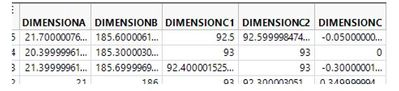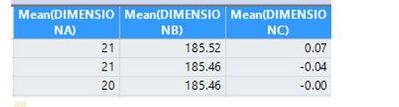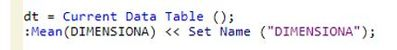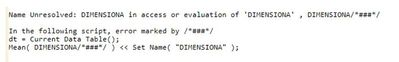Our World Statistics Day conversations have been a great reminder of how much statistics can inform our lives. Do you have an example of how statistics has made a difference in your life? Share your story with the Community!
Choose Language Hide Translation Bar
Highlighted

## How to Change a COL name of a SUMMARIZED table

Hello JMP community,

I would like to know how can I rename a column name after executing the "SUMMARY" function in JMP.

My raw data table is as shown:and I would require to Summarized this (get the average) and I will have the following data table:Now for my final table, I would want to rename the following:

Mean(DIMENSIONA) to "DIMENSIONA" only ,

Mean(DIMENSIONB) to "DIMENSIONB" only,

Mean(DIMENSIONC) to "DIMENSIONC" only and so on...

Im using the following code to do it but no to avail..

I am getting the following log. I dont know the work around, seems like the "Mean" is a function word so that's why I get this kind of error.I hope someone can help find solution or do anything about it. Much appreciated everyone's help!

1 ACCEPTED SOLUTION

Accepted Solutions
Highlighted

## Re: How to Change a COL name of a SUMMARIZED table

You will not have to rename the columns in the summary output table if you use the

statistics column name format( "column" )

option in the Summary Platform.  This is also a selection in the interactive dialog box on the lower left side, in an area titled "statistics column name format:

Here is a simple script illustrating what I am saying

``````Names Default To Here( 1 );
dt = Open( "\$SAMPLE_DATA\Fitness.jmp" );

dtSum = dt << Summary(
Mean( :Age ),
Mean( :Weight ),
Mean( :Oxy ),
Mean( :Runtime ),
Mean( :RunPulse ),
Mean( :RstPulse ),
Mean( :MaxPulse ),
Freq( "None" ),
Weight( "None" ),
statistics column name format( "column" ),
Link to original data table( 0 )
);``````

Jim
2 REPLIES 2
Highlighted

## Re: How to Change a COL name of a SUMMARIZED table

You will not have to rename the columns in the summary output table if you use the

statistics column name format( "column" )

option in the Summary Platform.  This is also a selection in the interactive dialog box on the lower left side, in an area titled "statistics column name format:

Here is a simple script illustrating what I am saying

``````Names Default To Here( 1 );
dt = Open( "\$SAMPLE_DATA\Fitness.jmp" );

dtSum = dt << Summary(
Mean( :Age ),
Mean( :Weight ),
Mean( :Oxy ),
Mean( :Runtime ),
Mean( :RunPulse ),
Mean( :RstPulse ),
Mean( :MaxPulse ),
Freq( "None" ),
Weight( "None" ),
statistics column name format( "column" ),
Link to original data table( 0 )
);``````

Jim
Highlighted

## Re: How to Change a COL name of a SUMMARIZED table

Thanks a lot! Works perfectly!
Article Labels

There are no labels assigned to this post.